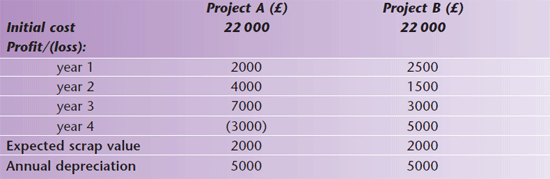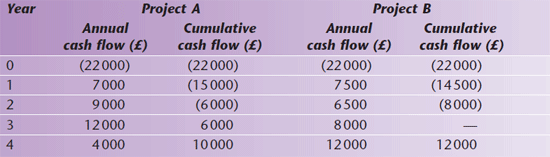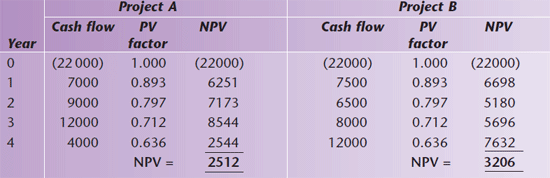# Investment Decision-making

Quick revise

Investment refers to the purchasing of productive capacity or capital expenditure (spending by a business to buy fixed assets e.g. property, vehicles etc)

Why Invest?

• Businesses need to invest to grow
• They might want to increase capacity so they can produce more
• If they produce more then they can sell more and increase sales revenue
• They could also look to invest to increase the efficiency of their operations

Because of the major capital outlay involved, managers try to calculate expected profitability and expected cash flows for the proposed investment. They use three methods of investment appraisal.

Payback period method
This method of investment appraisal calculates how long it takes a project to repay its original investment. The method therefore concentrates on cash flow, highlighting projects that recover quickly their initial investment. Here is an example of how it works.

A company is considering two different capital investment projects. Both are expected to operate for four years. Only one of the projects can be financed.The payback periods are calculated by adding annual depreciation back to the cash flows: depreciation is a non-cash expense that reduces profit, and so the profit figures given understate the cash inflows by the amount of the depreciation. Cash flow in year 4 is also increased by the scrap values for each project.The payback period for project A is two and a half years. At the start of year 3, outflows exceed inflows by £6000. Net inflows for year 3 are £12 000 (£1000 per month): it therefore recoups its original investment after six months of year 3.

Project B’s payback period is three years. The manager would therefore select project A on the basis of this method, even though project B generates a greater total cash inflow by the end of its life.

These calculations also help decisions to be made between alternative capital projects. Although the payback method is widely used in practice, it is often as a supplement to the other, more sophisticated, appraisal methods.

This method is easy to calculate and understand.

• Its use emphasises liquidity, because calculations are based solely on cash flow.
• It also helps managers to reduce risk by selecting the project that recovers its outlay most quickly.
• Early cash flows can be predicted more accurately than later ones, and are less affected by inflation.

The main drawback of this method is that it completely ignores profit and profitability. It also takes no account of interest rates.

Accounting rate of return (ARR) method
This method of investment appraisal calculates the expected profits from the investment, expressing them as a percentage of the capital invested. The higher the rate of return, the ‘better’ (i.e. the more profitable) is the project. The ARR is therefore based on anticipated profits rather than on cash flow.

ARR = (expected average profits / original investment) x 100

Using the above figures, project A generates total profits of £10 000 and project B total profits of £12 000.

 Project A (£) Project B (£) 2500 3000 22000 22000 APR = 11.4% APR = 13.6%

Project B would therefore be selected using ARR.

• The accounting rate of return method is easy to use and simple to understand.
• It measures and highlights the profitability of each project.

Its disadvantages are that it ignores the timing of a project’s contributions. High profits in the early years – which can be estimated more accurately, and which help minimise the project’s risk – are treated in the same way as profits occurring later. It also concentrates on profits rather than cash flows, ignoring the time value of money (profits in the later years being eroded by the effects of inflation).

Discounted cash flow (DCF) method
The principle of DCF is based on using discounted arithmetic to get a present value for future cash inflows and outflows. This method is sometimes divided into two elements, which complement each other:

• the net present value (NPV) method, which takes account of all relevant cash flows from the project throughout its life, discounting them to their ‘present value’
• the internal rate of return (IRR) method, which compares the rate of return expected from the project with that identified by the company as being the cost of its capital – projects having an IRR that exceeds the cost of capital are worth considering.

As an example, a company receiving £100 at the start of a year might be able to invest it at 10% per annum: by the end of the year this investment will be worth £110. Given the choice of £100 now or a higher sum in a year’s time, the managers will choose the higher sum only if it exceeds £110. This principle works in reverse: the managers know that a project generating £110 in a year’s time is worth the same as one generating £100 immediately, the project with the future value being discounted to its present value (by using a set of discounting tables).

The NPV method calculates the present value of the project’s future cash flows. Each year’s cash flow is discounted to a present value, which shows how much the managers would have to invest now at a given rate of interest to earn these future cash benefits. The present value of the total cash outflows is compared to that of the total cash inflows to calculate the net present value of the project. The project with the highest positive NPV will be chosen.

• If the NPV is positive – cash benefits exceed cash costs – this means that the project will earn a return in excess of its cost of capital (the rate of interest/discounting used in the calculations).
• If the NPV is negative, this means the cost of investing in the project exceeds the present value of future receipts, and so it is not worth investing in it.

If the company planning to invest in either project A or B has a cost of capital equal to 12%, the future cash flows can be discounted to their present values using discounting tables. The present value of £1 when discounted at 12% is:

 Year Present value (PV) factor 12% 0 1.000 1 0.893 2 0.797 3 0.712 4 0.63Project B has the higher expected NPV and would therefore be selected. The IRR method involves comparing the actual rates of return – in this illustration, both rates exceed 12% since both show positive NPVs when discounted at 12% – with the company’s cost of capital.

The use of DCF takes account of all cash flows, and it acknowledges the time value of money. The main problem is in establishing a suitable discount rate to use, because this rate (and the firm’s cost of capital) is likely to vary over the life of the project.

KEY POINTS - Organisations undertake investment appraisal to evaluate the likely success and value of capital investments, which tie up the firm’s finance for a long period, normally for a number of years.